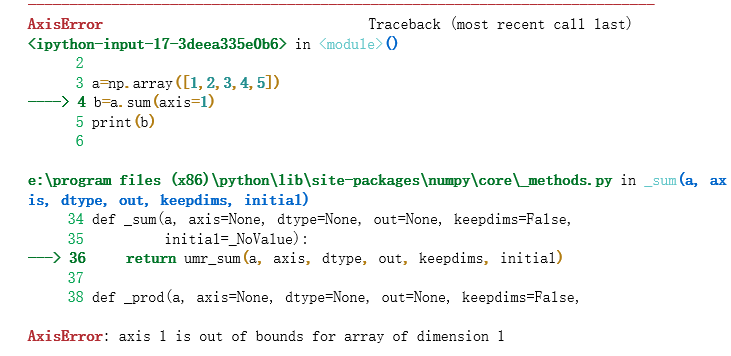• 2020-12-28 21:44:20

python编写一个函数my_sum1，其功能是根据给定的数N求得从1到N间所有数的和并返回

# 包含N

def my_sum1(N):

return sum([x for x in range(1, N 1)])

print my_sum1(10)

python sum函数怎么写

sum是python中很实用的函数，但是要它的使用，我第一次用的时就把它这了：

s

=

sum(1,2,3)

结果就悲剧啦

其实sum()的参数是一个list

例如：

sum([1,2,3])

sum(range(1,11))

还有一个比较有意思的用法

a

=

range(1,11)

b

=

range(1,10)

c

=

sum([item

for

item

in

a

if

item

in

b])

print

c

输出：

45

求问python 2 中的print sum()在python 3 中要如何表达，sum()为函数，谢谢。

搜一下：求问python

2

中的print

sum()在python

3

中要如何表达，sum()为函数，谢谢。

python的sum函数怎么用？

sum(iterable[, start]) ，iterable为可迭代对如：

sum([ ], start)  ， #iterable为list列表。

sum(( ), start ) ， #iterable为tuple元组。

的值=可对应里面的数相加的值 start的值

start默认为0，如果就是0，为0时可以不写，即sum()的参数最多为两个，其中第一个必须为iterable。

版权声明：本站所有文章皆为原创，欢迎转载或转发，请保留网站地址和作者信息。

更多相关内容
• ## pythonsum函数的用法

万次阅读 2018-12-27 10:40:20
需要用到python sum（）函数，总结了一下，不对之处烦请指出，谢谢。 1维情况下  （1）输入axis=0 import numpy as np a=np.array([1,2,3,4,5]) b=a.sum(axis=0) print(b)  输出结果：15, 也就是一维数组...

需要用到python sum（）函数，总结了一下，不对之处烦请指出，谢谢。

# 1维情况下

## （1）输入axis=0

import numpy as np

a=np.array([1,2,3,4,5])
b=a.sum(axis=0)
print(b)

输出结果：15,   也就是一维数组各个元素的和

## （2）如果在一维情况下输入axis=1则会报如下错误，也就相当于数组维度越界。# 2维情况下

## (1)输入axis=0

import numpy as np

b=np.array([[3,7,6],[2,4,5]])
print(b.sum(axis=0))

相当于是：
[[3,7,6],
[2,4,5]]
没一列对应元素相加
3+2,7+4,6+5
输出：
[ 5 11 11]

此时相当于是将每一列对应的元素相加，即3+2=5,7+4=11,6+5=11

## （2）输入axis=1

import numpy as np

b=np.array([[3,7,6],[2,4,5]])
print(b.sum(axis=1))

输出为
[16 11]
相当于是3+7+6，2+4+5，即每一行的元素分别相加

相当于是3+7+6，2+4+5，即每一行的元素分别相加

# 3维情况下

## （1）axis=0

import numpy as np

b=np.array([
[[3,7,6],[3,4,5]],
[[2,4,5],[1,1,1]]
])
print(b.sum(axis=0))
输出[[ 5 11 11]
[ 4  5  6]]
类似二维的情况[[3+2,7+4,6+5],[3+1,4+1,5+1]]

## (2)axis=1

import numpy as np

b=np.array([
[[3,7,6],[3,4,5]],
[[2,4,5],[1,1,1]]
])
print(b.sum(axis=1))

输出[[ 6 11 11]
[ 3  5  6]]
三维中包含两行两列的二维数组，每一行对应的元素分别相加【[3+3,7+4,6+5],[2+1,4+1,5+1]】
以结果的第一行为例，结果由来是：
[
[ b+b, b+b, b+b ],
[ b+b, b+b, b+b ]
]

## (3)axis=2

import numpy as np

b=np.array([
[[3,7,6],[3,4,5]],
[[2,4,5],[1,1,1]]
])
print(b.sum(axis=2))

输出[[16 12] ------>3+7+6,3+4+5
[11  3]------>2+4+5，1+1+1]


展开全文• 一.Python sum 函数介绍 二.Python sum 函数使用 三.猜你喜欢 基础 Python 学习路线推荐 : Python 学习目录 >> Python 基础入门 一.Python sum 函数介绍 sum 函数作为 Python 内置函数，顾名思义，可以对...

目录

基础 Python 学习路线推荐 : Python 学习目录 >> Python 基础入门

## 一.Python sum 函数介绍

sum 函数作为 Python 内置函数，顾名思义，可以对迭代器中的所有元素求总和，语法如下：

'''
参数介绍：
iterable — 可迭代对象，如：列表、元组、集合；
start — 指定相加的参数，如果没有设置这个值，默认为0；

返回值 — 返回迭代器中所有元素相加得总和；
'''
sum(iterable,start=0)


## 二.Python sum 函数使用

# !usr/bin/env python
# !usr/bin/env python
# -*- coding:utf-8 _*-
"""
@Author:猿说编程
@Blog(个人博客地址): www.codersrc.com
@File:Python sum函数.py
@Time:2021/04/18 07:37
@Motto:不积跬步无以至千里，不积小流无以成江海，程序人生的精彩需要坚持不懈地积累！

"""

print(sum([0,1,2]))    # 等价 0 + 1 + 2 = 3
print(sum((0,1,2),10)) # 等价 0 + 1 + 2 + 10 = 13
print(sum([0,1,2],20)) # 等价 0 + 1 + 2 + 20 = 23

'''
输出结果：

3
13
23
'''


## 三.猜你喜欢

未经允许不得转载：猿说编程 » Python sum 函数

展开全文• ## pythonsum函数怎么用

千次阅读 2021-01-28 19:02:53
之前误用sum()函数，我又去查了查python sum()函数才恍然大悟。我本来想算几个Int值相加的和，本以为很简单的事情，结果却很悲伤，例：>>>sum=sum(1,2,3)#结果很明显出现问题报错TypeError:...之前误用sum()函数，我又去查了查python sum()函数才恍然大悟。

我本来想算几个Int值相加的和，本以为很简单的事情，结果却很悲伤，例：>>>sum = sum(1,2,3)         #结果很明显出现问题报错

TypeError: sum expected at most 2 arguments, got 3

傻乎乎的我以为只能算前两个数的和等于3，就又试了一下>>>sum = sum(1,2)           #结果还是报错

TypeError: 'int' object is not iterable

其实，我们所了解sum()函数语法是这样的sum(iterable[, start])

其中

iterable – 可迭代对象，如：列表(list)、元组(tuple)、集合(set)、字典(dictionary)。

start – 指定相加的参数，如果没有设置这个值，默认为0。

也就是说sum()最后求得的值 = 可迭代对象里面的数加起来的总和(字典:key值相加) + start的值(如果没写start的值，则默认为0)>>>sum = sum([1,2,3])             # in list

6

如果我们加上start的话，应该是这样的>>> sum = sum([1,2,3],5)          #in list +start

9

>>> sum = sum((1,2,3))            #in tuple

6

>>> sum = sum({1,2,3})            #in set

6

>>> sum = sum({1:5,2:6,3:7})      #in dictionary key

6

>>> sum = sum(range(1,4)          #in range()

展开全文• ## Pythonsum()函数

千次阅读 2022-02-20 16:58:22
Python里的sum函数语法例子1. 列表中的元素为数字：2. 列表中的元素为字符串：3. 列表中元素为列表 语法 sum(iterable, start) 参数1 iterable，一个可迭代对象，可以是列表、字典、元祖等，重要的是可迭代对象中的...
• sum(iterable[, start]) ，iterable为可迭代对象，如：sum([ ], start) #iterable为list列表sum(( ), start ) #iterable为tuple元组......最后的值 = 可迭代对象里面的数相加的值 + start的值start默认为0，如果不写...
• 注意：iterable – 可迭代对象...sum=iterable的和+start的值 1、列表 print(sum([1, 2])) print(sum([1, 2], 3)) 3 6 2、元组 print(sum((1, 2))) 3 3、字典（只对键相加） print(sum({1: 3, 2: 3})) 3 ...开发语言 后端
• ## python中sum函数详解

千次阅读 2019-09-11 14:48:38
python内置的sum函数 对象是列表，元组，集合，可以混合起来使用 >>>sum([1,2,3],2) 8 >>>sum((1,2,3)) 6 >>>sum({1,2,3}) 6 >>>sum(range(5)) 10 numpy中sum...
• ## python求和函数sum()详解

万次阅读 多人点赞 2019-04-06 21:32:44
python求和函数sum()详解 今天在学习的过程中，误用sum()函数，我又去查了查python sum()函数才恍然大悟。 我本来想算几个Int值相加的和，本以为很简单的事情，结果却很悲伤，例： >>>sum = sum(1,2,3) #...
• 一:python3中内置的sum函数用法:sum(iterable, start)iterable:可迭代对象(如列表,元组,集合)start:指定相加的参数,如果没有设置这个值,默认为0返回一个指定相加的参数即stardt(默认为0)加上一个数字的可迭代对象的...
• sum(iterable[, start]) ，iterable为可迭代对象，如：sum([ ], start) ， #iterable为list列表。sum(( ), start ) ， #iterable为tuple元组。最后的值=可迭代对应里面的数相加的值 + start的值start默认为0，如果不...
• 今天小编就为大家分享一篇基于Python中求和函数sum的用法详解，具有很好的参考价值，希望对大家有所帮助。一起跟随小编过来看看吧
•机器学习 算法 numpy
• sum函数是对系列求和的函数 1.语法： sum(iterable[, start]) 2.参数 iterable -- 可迭代对象，如：列表、元组、集合。 start -- 指定相加的参数，如果没有设置这个值，默认为0。 3.实例 ...
• ## sum函数语法与使用

千次阅读 2020-11-25 13:40:15
一、sum 函数语法：SELECT SUM(expression ) FROM tables WHERE predicates;expression常量、列或函数，或者是算术、按位与字符串等运算符的任意...二、使用SUM函数需要注意以下几点：（1）含有索引的字段能够加快聚...
• Python内置的sum函数 笔者的理解 拓展：NumPy中sum函数 求相似度时看到的，碰到一个疑问的地方，先写下来。 《机器学习基础：相似度和距离度量究竟是什么》 Python内置的sum函数 作用：对可迭代对象进行求和...
• def my_sum(lst): sum_res = 0 if not isinstance(lst,list): return sum_res for item in lst: if isinstance(item,(float,int)): sum_res = sum_res + item return sum_res if __name__ == '__main__': ...
• sumpython中一个很实用的函数，但是要注意它的使用，我第一次用的时候，就把它这样用了： 1 s=sum(1,2,3) 结果就悲剧啦 其实sum()的参数是一个list 例如： 1 2 sum(...开发语言 后端
• python中的sum()函数用来进行求和计算，函数语法为：sum(iterable[,start])，此函数的返回值为计算的结果。 函数参数有：（大白话就是括号里面的相加，外面的数相加几就写几，不写默认为零） iterable -- 可迭代...
• answer+=sum(S.count(i) for i in J) S、J都是字符串 ...我明白这句的意义，理解能完成的功能，但是找不到这种（for写在sum函数写法）的出处，不理解的点是for写在这个位置为什么会影响前面S.count(i)
• ## python中 sum() 函数

千次阅读 2019-03-02 11:32:20
sum( axis = 0 )，列的相加； sum( axis = 1 )，行的相加； sum()，总的相加； &gt;&gt;&gt; c = array([[1,2, 1], [2, 1, 0], [3,2,1]]) &gt;&gt;&gt; c array([[1, 2, 1], [2, 1, 0], ...
• 使用Numpy(下面简称np)中的sum函数对某一维度求和时，由于该维度会在求和后变成一个数，所以所得结果的这一维度为空。比如下面的例子：a = np.array([[1,2,3],[4,5,6]])b = np.sum(a,axis=1)print(b.shape)# (2,)...
• ## Python中的sum函数一个妙用

万次阅读 多人点赞 2018-04-14 10:59:16
这个时候使用Python自带的sum函数会很简单。 先看下sum函数的帮助文档:从上面的帮助文档可以看出:sum函数是个内置函数，可以求一个数字列表的和，并且可以带初始值，如果不带初始值的话，默认是0.比如说：&gt;&...list start
• [root@steve-lidiliang /user/MLiA_SourceCode/Ch02]# python Python 2.7.5 (default, Nov 6 2016, 00:28:07) [GCC 4.8.5 20150623 (Red Hat 4.8.5-11)] on linux2 Type "help", "copyright",...
• Python自带的sum函数与numpy中的sum函数有着天壤之别，没弄懂之前踩了大坑。 1、Python 自带的sum Python自带的sum输入是个可迭代的。可以是列表，数组，可迭代对象。此时sum最多有两个参数第一个参数是可迭代的。...
• 代码来自《Python数据分析基础教程NumPy学习指南（第二版）》中第...代码中定义函数pythonsum有错误：应为如下 def python_sum(n): a = list(range(n)) b = list(range(n)) c = [] for i in range(len(a)): ...sys.argv
• 定义一个累加求和函数sum1(n),函数代码如下： 2、使用 for循环 定义一个累加求和函数sum2(n),函数代码如下： 3、使用递归函数 定义一个累加求和函数sum3(n),函数代码如下： 二、使用了三种实现累加求和的方法，......